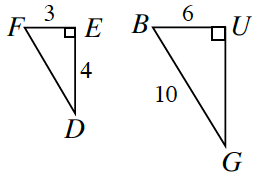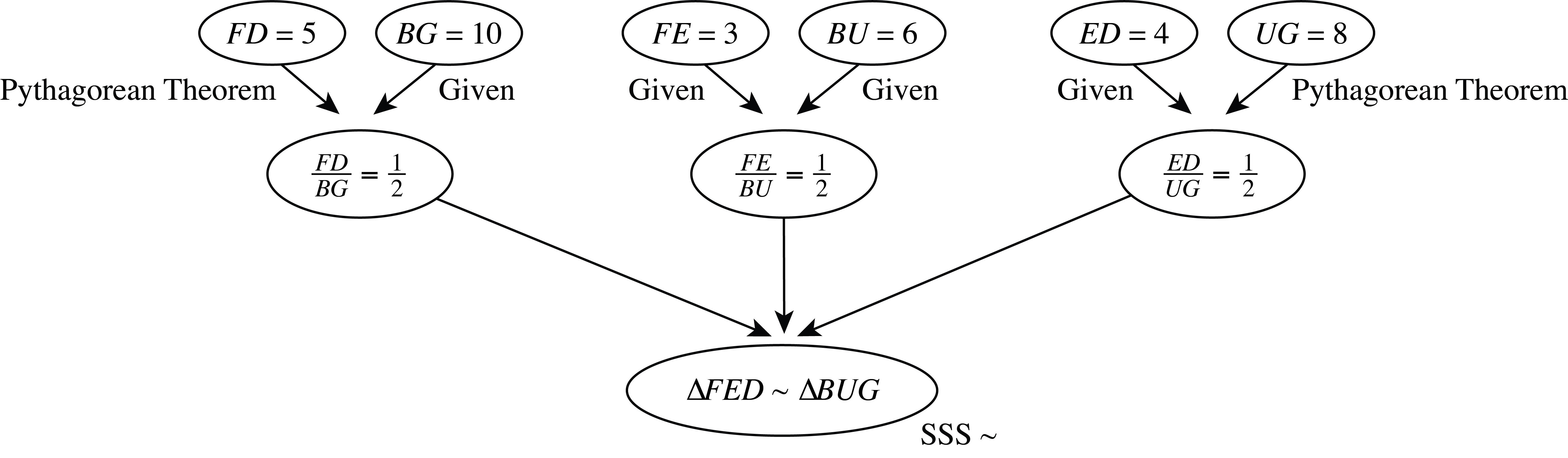### Home > CCG > Chapter 7 > Lesson 7.1.4 > Problem7-46

7-46.

For each pair of triangles below, determine whether or not the triangles are similar. If they are similar, show your reasoning in a flowchart. If they are not similar, explain how you know.

1.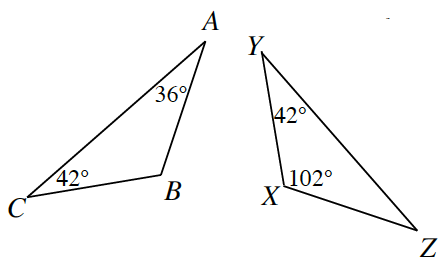measure of Angle YXZ = 102 degrees,  measure of angle BAC = 36 degrees, and  Angle ACB is congruent to angle ZYX.

measure of Angle CBA = 102 degrees and measure of Angle XZY = 36 degrees, both by the Triangle Angle Sum Theorem.

All three angles are the same in both triangles.

Triangle A, B, C is similar to triangle X, Y, Z by A, A similarity.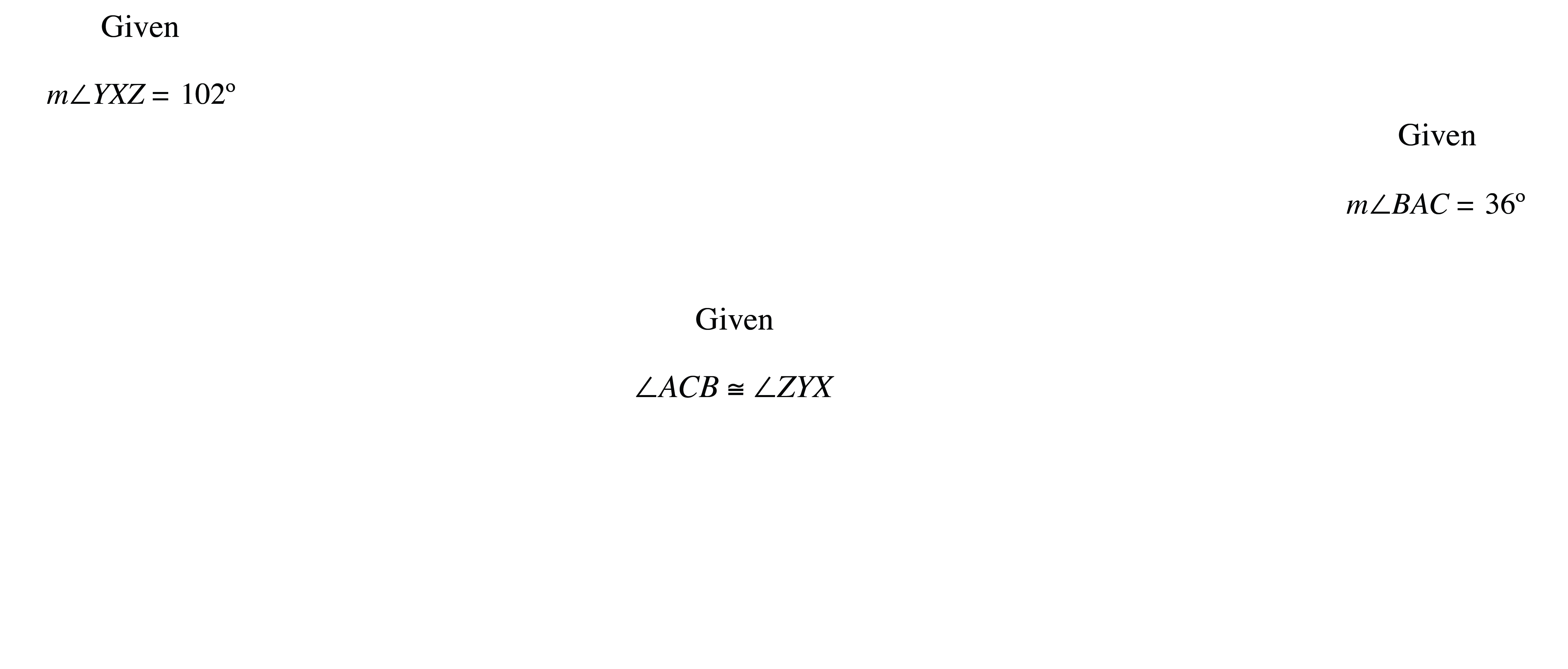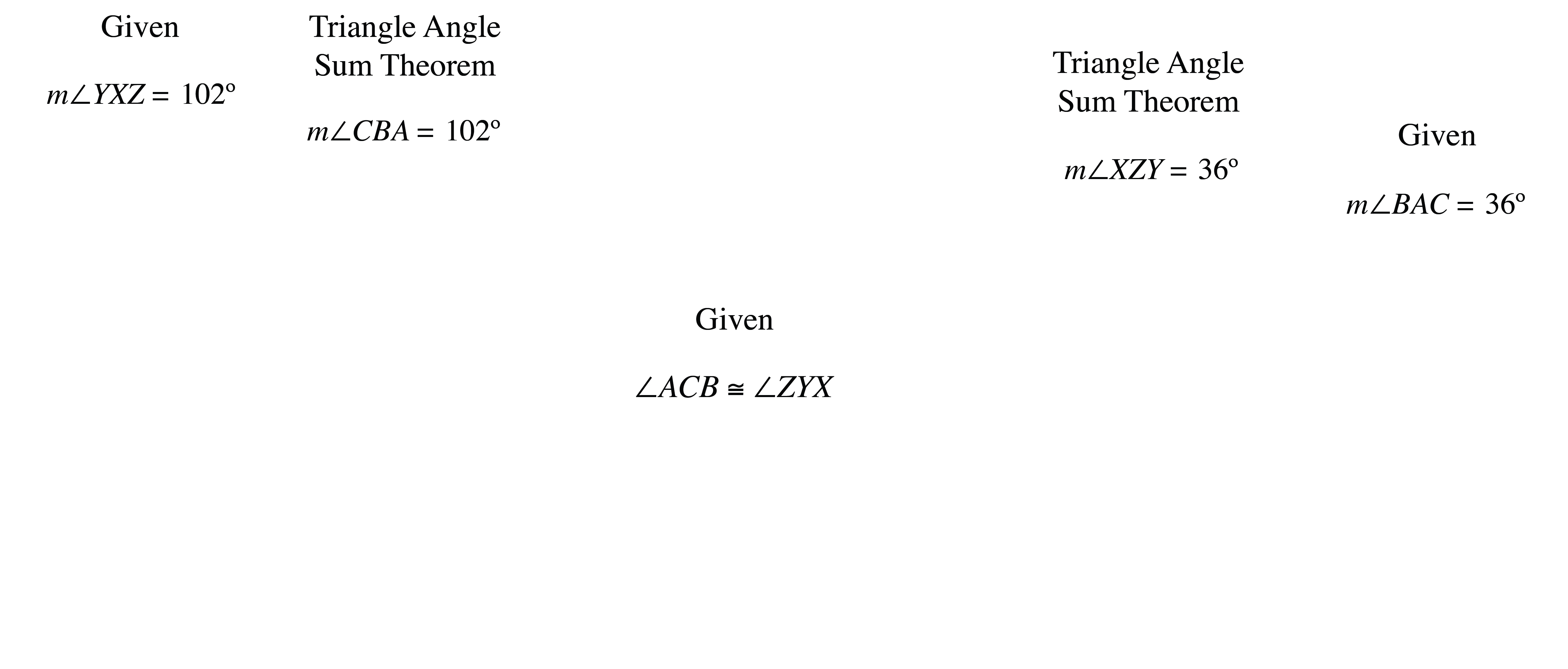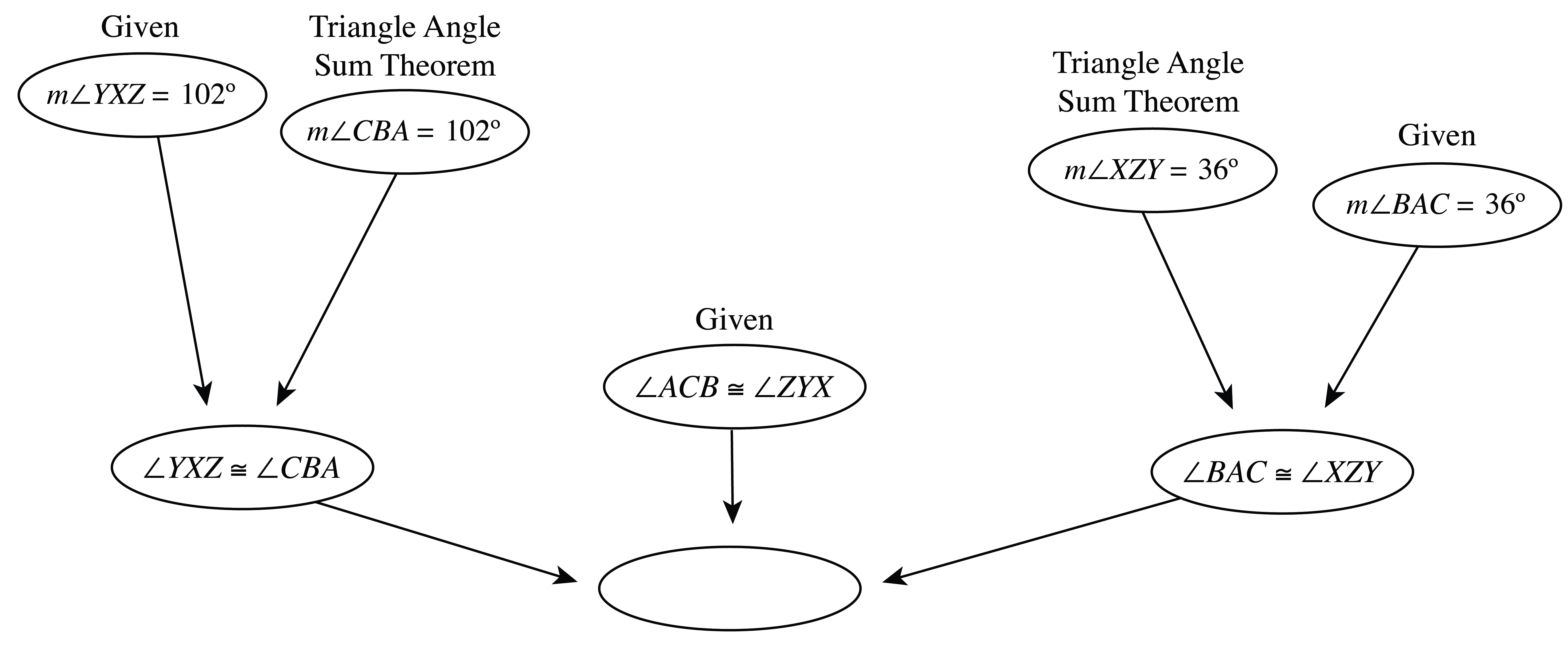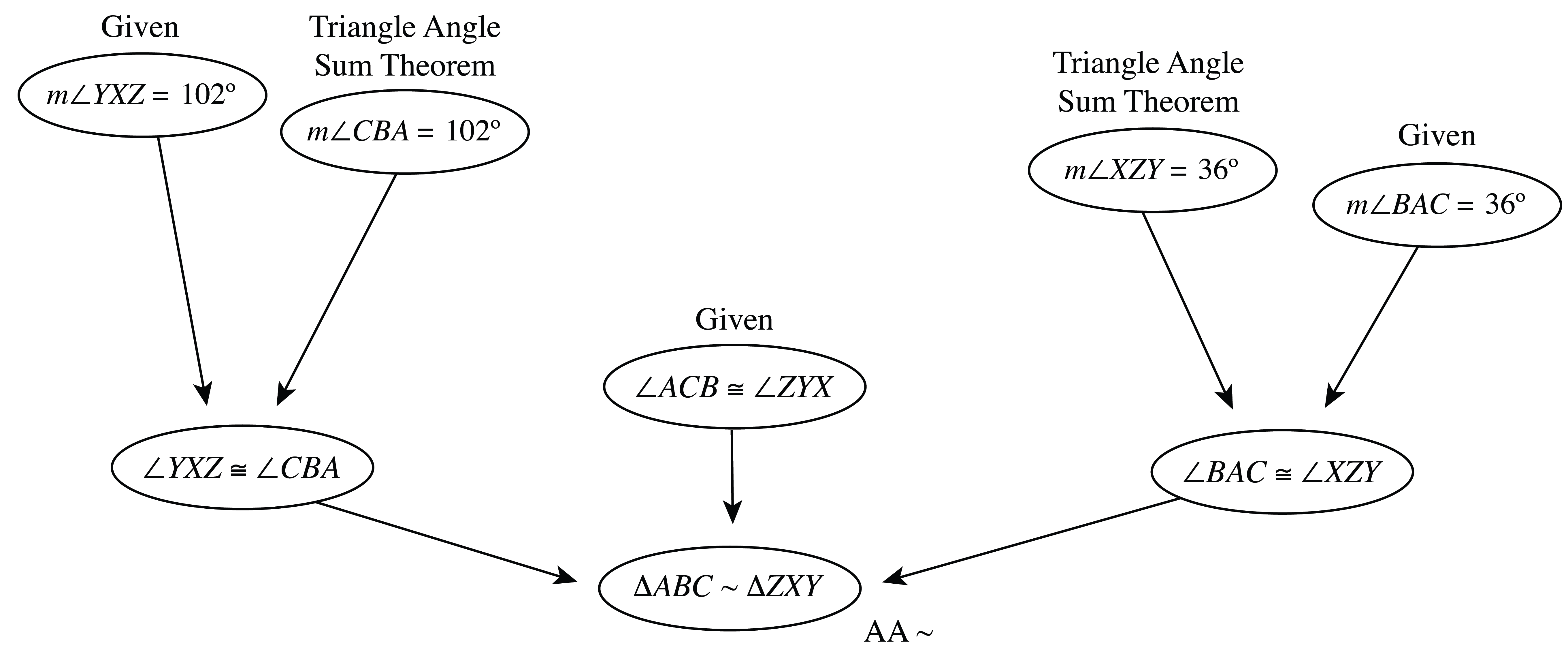2.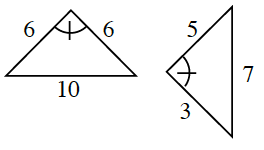The triangles are not similar because corresponding sides do not have the same ratio. One triangle is isosceles and the other is not.

3.